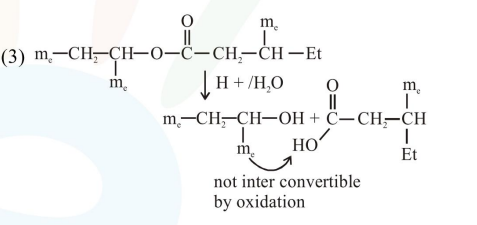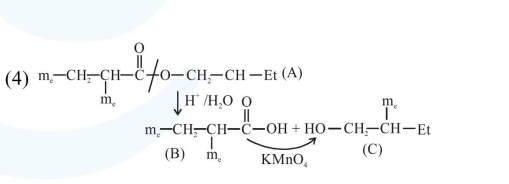# An organic compound

Question:

An organic compound [A], molecular formula $\mathrm{C}_{10} \mathrm{H}_{20} \mathrm{O}_{2}$ was hydrolyzed with dilute sulphuric acid to give a carboxylic acid [B] and alcohol [C]. Oxidation of [C] with $\mathrm{CrO}_{3}-\mathrm{H}_{2} \mathrm{SO}_{4}$ produced [B]. Which of the following structures are not possible for $[\mathrm{A}]$ ?

1. $\left(\mathrm{CH}_{3}\right)_{3}-\mathrm{C}-\mathrm{COOCH}_{2} \mathrm{C}\left(\mathrm{CH}_{3}\right)_{3}$

2. $\mathrm{CH}_{3} \mathrm{CH}_{2} \mathrm{CH}_{2} \mathrm{COOCH}_{2} \mathrm{CH}_{2} \mathrm{CH}_{2} \mathrm{CH}_{3}$

3.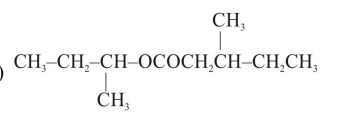4.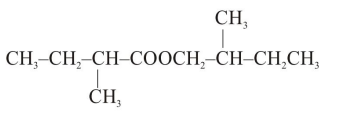Correct Option: , 3

Solution: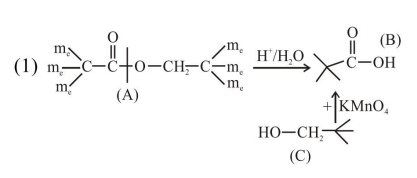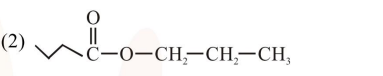Total $8^{\prime} \mathrm{C}^{\prime} \rightarrow$ so molecular formula not matched.